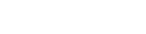# JavaScript Array map()

You may use the Array.map() method to traverse over an array and alter its elements via a callback function. The callback function will then be run on each element of the array. A Map object also retains the order in which the keys were first inserted. The Map object stores key-value pairs in which any type of value may be used as either a key or a value. Lets imagine we want to multiply our array by 5. Lets see how we can achieve this with a for loop first.

## Syntax

``````
array.map(function(currentValue, index, arr), thisValue)

``````
ParameterDescription
function()For each array element, a function must be executed.
currentValueThis is the current elements value.
indexThe current elements index number.
arrThis is the current elements array.
thisValueA value that will be used by the function. The default value is undefined.

## Using for loop

``````
let array = [1, 2, 3, 4, 5];

for (let i = 0; i < array.length; i++){
array[i] = array[i] * 5;
}

console.log(array);

``````
##### Output
``````
[ 5, 10, 15, 20, 25 ]

``````

Lets see how we can iterate over an array using map() method.

## Using map method

``````
let array = [1, 2, 3, 4, 5];

let result = array.map(function(element){
return element * 5;
});

console.log(result);

``````
##### Output
``````
[ 5, 10, 15, 20, 25 ]

``````

Lets see how we can iterate objects over an array using map() method.

## Using map method for objects

``````
let employee = [
{empID : "101", empName: "Vishal"},
];

let empInfo = employee.map(function(element){
return [element.empID,element.empName];
})

console.log(empInfo);

``````
##### Output
``````
[ [ '101', 'Vishal' ],

``````

Lets see how we can use function for multiplying

``````
const no = [1, 2, 3, 4, 5];
const array = no.map(multiply)
console.log(array)

function multiply(num) {
return num * 5;
}

``````
##### Output
``````
[ 5, 10, 15, 20, 25 ]

``````